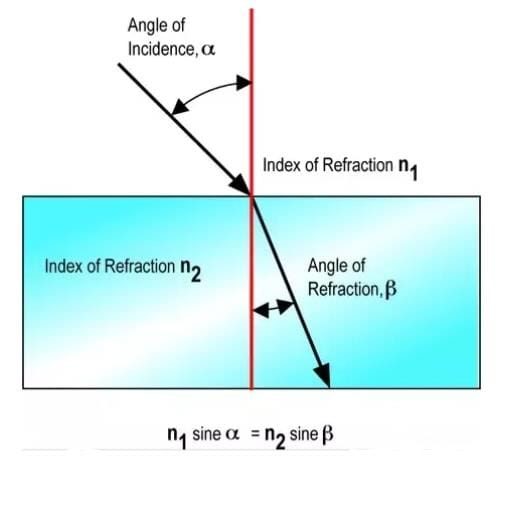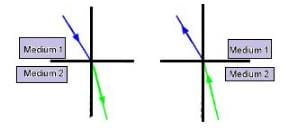# Test: Refraction by Plane Surfaces

## 10 Questions MCQ Test Physics For JEE | Test: Refraction by Plane Surfaces

Description
Attempt Test: Refraction by Plane Surfaces | 10 questions in 10 minutes | Mock test for NEET preparation | Free important questions MCQ to study Physics For JEE for NEET Exam | Download free PDF with solutions
QUESTION: 1

### Just before setting, the sun may appear to be elliptical. This happens due to:

Solution:

Refraction of light ray through the atmosphere may cause different magnification in mutually perpendicular directions.

Sunlight enters the Earth's atmosphere from the vacuum of space. The refractive index of air with respect to vacuum is 1.0029. This means that the speed of light in air is 1.0029 times slower than the speed of light in vacuum. This causes the light rays to bend towards the normal (refraction).QUESTION: 2

### Bending effect of light as it passes from one transparent material into other is known as

Solution:

This 'bending of a ray of light' when it passes from one substance into another substance is called refraction. The bending of a ray of light also occurs when the ray comes out of glass or water and passes into air.

QUESTION: 3

### An air bubble in glass slab (μ=1.5) from one side is 6 cm and from other side is 4 cm. The thickness of glass slab is :

Solution:

We know that μ=(apparent depth/real depth​)

Let the thickness of the slab be t and real depth of the bubble from one side be x. Then

μ=(x/6)​=(t−x)/4​ or 1.5=x/6​ = (t−x​)/4

This gives x=9 and 1.5=(t−9)/4​ or t=15cm

QUESTION: 4

What is the Principle of Reversibility?

Solution:

The principle of reversibility of light states that light follows the same path if the direction of the travel of light is reversed.
The principle of reversibility presumes that the attenuation of a light ray during its passage through an optical medium because of reflection, refraction, and absorption is not affected by a reversal of the direction of the ray. In its simplest explanation, the principle of reversibility is a deduction of Snell’s law of the refraction of light. The principle of reversibility presumes that the attenuation of a light ray during its entrance through an optical medium due to reflection, refraction, and absorption is not changed by a reversal of the direction of the ray.QUESTION: 5

A microscope is focused on an ink mark on the top of a table. If we place a glass slab 3cm thick on it, how should the microscope be moved to focus the ink spot again? The refractive index of glass slab is 1.5 cm.​

Solution:

The image of the ink spot moves up by 1cm.

Refractive index = Real depth/ apparent depth

1.5 = 3/ apparent depth

Apparent depth = 3/1.5 = 2 cm

Distance through which the microscope is moved is 3 - 2 cm = 1 cm upwards

QUESTION: 6

Can absolute value of refractive index be less than unity?

Solution:

Absolute refractive index of a medium = speed of light in vacuum / speed of light in in that medium.

Since the speed of light is maximum in vacuum, the refractive index cannot be less than unity.

QUESTION: 7

When light undergoes refraction, its frequency

Solution:

Light refracts as it passes through a material. It's direction changes, but it still passes through. Light travels at a maximum speed -- the speed of light in a vacuum, but when traveling in anything else it slows down. Different materials slow the speed of passing light at different rates. This property of matter is called the refractive index. When refracting, light doesn't change it's frequency, but since it changes it's speed, it must also change it's wavelength (it gets squished or elongated). Frequency, wavelength, and speed are all related, so if one property changes, another must as well.

QUESTION: 8

A ray of light passes through a plane glass slab of thickness t and refractive index μ = 1.5. The angle between the incident ray and emergent ray will be:​

Solution:

The incident ray and emergent ray are parallel to each other but latteray displaced due to reflaction at two surfaces. So, the angle between them is Zero.

QUESTION: 9

When light undergoes refraction, the wavelength

Solution:

Light is refracted when it crosses the interface from air into glass in which it moves more slowly. Since the light speed changes at the interface, the wavelength of the light must change, too. The wavelength decreases as the light enters the medium and the light wave changes direction

QUESTION: 10

Due to _______ the depth of an optically denser medium appears to be _______ than its real depth.

Solution:

When light moves from 1 medium to another, refraction takes place. And when light enters a denser medium, the ray bends away from normal and meets at a point above the actual point where they would have met, if the medium was absent. So, apparent depth decreases.Use Code STAYHOME200 and get INR 200 additional OFF Use Coupon Code